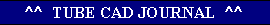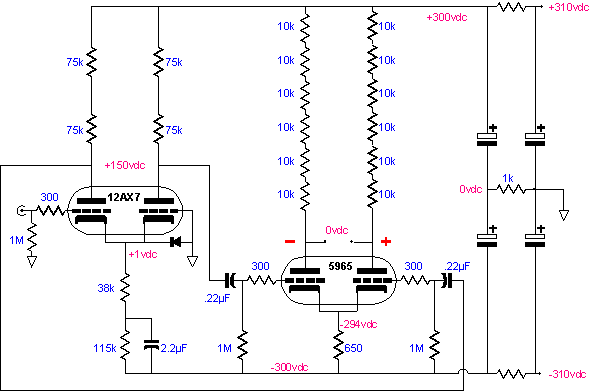By making the cathode resistor equal to the value of the two plate resistors in parallel, the power supply noise at the plates of the 12AX7 nulls out. By making the cathode resistor equal to one half the value of the two plate resistors in parallel, the power supply noise at the plates of the 12AX7 matches the negative rail noise, in both phase and amplitude. This last scenario is our answer.  Of course, this value of cathode resistor resistance is not sustainable in this circuit, as its direct implementation would quadruple the current flow through the 12AX7, as 300 volts divided by 37.5k equals 8 mA. Indirectly used, however, the problem disappears. A string of zener diodes with a break voltage of 225 volts would work, but the noise… In this case the two 150k plate resistors in parallel equal 75k, which if halved, equals 37.5k. (And 37.5k in series with a capacitor bypassed 122.5k resistor equals the needed 150k to bias the idle current through the 12AX7 perfectly. Yes, 37.4k and 113k will work just fine.)
 With this modification to the circuit, much of noise from just one of either output decreases markedly. So much so that the feedback loop and the two small valued resistors at the 12AX7's cathodes were removed.   A second electrostatic headphone  amplifier was built using a 5751 for the first stage and a 5965 for the output. The 5965 was originally intended for tube computer use and in this position is a gem. It makes for a much higher total gain and allows for huge voltage swings.  The 60k plate resistors were replaced with six 10k resistors. There is a voltage distortion mechanism in many resistors that is so small at low voltages that it is usually disregarded, but in a high voltage circuit, such as this one, the distortion becomes an issue. Using six resistors in series reduces the resistor distortion by six fold. Additionally, if the wrong tube is accidentally plug into this amplifier, the plate resistors will survive the rail to rail voltage, as each resistor is rated for 1 watt. Always think of the worst case scenario.Second version of the all tube electrostatic headphone OTL amplifier with noise cancelling tricks.pg. 7